# Characterizing the Geometry of Monocrystalline Diamond Contouring Tools

Diamond cutting tools are highly accurate instruments that are used to create precisely contoured surfaces. Thanks to recent advancements in the development of these cutting tools, the geometry of the tool tip can now be produced within a few micro-inches of perfection.

The cutting tool geometry must be known to a similar degree of accuracy to create components that have contour accuracies of a few micro-inches. This article demonstrates a single technique through which the geometry of a diamond cutting tool can be completely defined, thereby making it possible to determine a tool path that would produce a predefined workpiece accurately.

Similarly to other cutting tools, diamond tools are usually defined by asserting a value for the tool radius. This value, together with the sweep angle, the clearance angle, and the top rake angle, are deemed to detect the properties of the tool. However, such data is not sufficient to characterize the tool accurately.

On a contouring tool, the clearance angle can be produced by creating a cylindrical surface on the tool nose, at an angle to the proposed cutting plane. Alternatively, the clearance angle can be formed by creating a conical surface with an axis perpendicular to the cutting plane. However, each method comes with certain advantages and disadvantages.

One benefit of a cylindrical clearance tool is that its radius remains unchanged when the upper face of the tool is relapped. Such tools are known to be more robust than conical clearance tools. Conversely, a major drawback of a cylindrical clearance tool is that the clearance angle reduces as the cut shifts away from the tool axis and ultimately approaches zero degrees. One advantage of a conical clearance tool is that the clearance angle does not change as the cut shifts away from the tool axis, but the main disadvantage is that the tool radius will change each time a conical clearance tool is relapped, and hence will need to be qualified again.

The radius of the tool holds major significance. A large number of diamond tools are elliptical instead of round. This is illustrated in Figure 1, which shows a zero degree top rake, cylindrical clearance tool with a clearance angle of 10°. In this standard configuration, it can be observed that the upper rake face of the tool lies in the cutting plane, crossing the clearance cylinder at 10° angle. This, in turn, creates an error in the tool radius of 0.000011 at a point 40° off the axis of the tool. Apart from the case where the top rake is equivalent to -1 times the clearance angle, the entire cylindrical rake tools are elliptical. Even in this example, when the tool’s cutting edge is projected into the cutting plane, an ellipse is formed by the tool.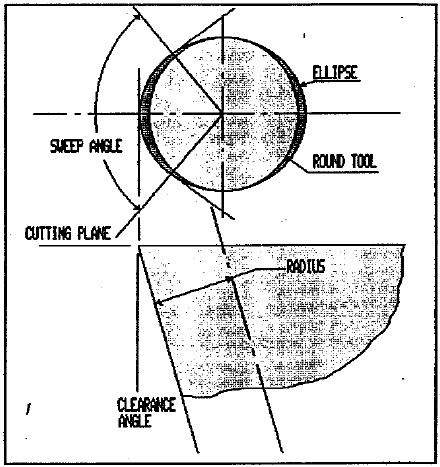Figure 1. Even a tool with a zero degree top rake is an ellipse which differs significantly from the circle which is commonly used to approximate it.

Conical clearance tools with a top rake of zero degrees are clearly circular, but conical clearance tools with a top rake equal to -2 times the clearance angle are also observed to be circular, even though their projection is also an ellipse when the cutting edge of the tool is projected into the cutting plane.

How should the tool radius be characterized? One standard method to determine the tool radius is to employ a diamond tool analyzer to turn the tool under a microscope, while at the same time adjusting the position of the microscope, as well as the position of the tool, in relation to the center of rotation. Initially, the tool’s edge is centered, followed by the center of the circle which optimally fits the edge. The variation between both these positions is the radius of the best-fit circle. This is the value which is specified as the tool "radius". After making this measurement, the tool is tipped so that its top rake surface remains in the plane of rotation. This ensures that the tool’s edge remains in focus across the entire range of rotation. The angle through which the tool was rotated must be considered along with the tool radius.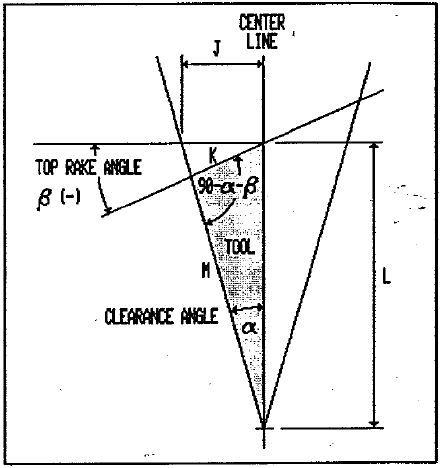Figure 2. A negative top rake tool with a conical clearance angle. The tool is shown as a cross section taken along its axis of symmetry.

With reference to Figure 2, which shows a negative rake, conical clearance tool, the length of line J (one axis of the ellipse) is as follows: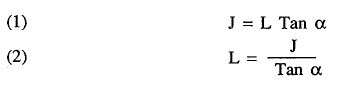From this, the other axis K can be stated as follows: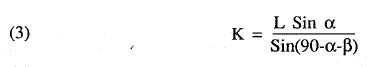The formula for the ellipse is: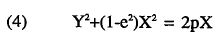Solving this expression for p: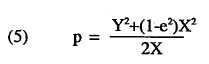The eccentricity of the ellipse, e is: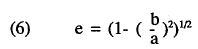In Equation 6, ‘a’ is the major axis of the ellipse and ‘b’ is the minor axis. By replacing K for ‘a’ and J for ‘b’, it was observed that the fraction a/b includes only functions of the angles a and ß. When equation 6 was evaluated, it was found that its value surpasses 1. It is known that J, rather than K, is the main axis of the ellipse. If this is the case, prior to solving Equation 6 for e, Equation 7 must be inverted so that its value is below 1.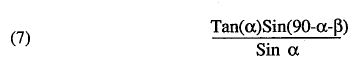This can be simplified as follows: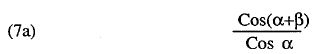To solve Equation 5, values for both X and Y must be acquired. These values arise from the best-fit circle, which was detected by means of the diamond tool analyzer. For instance, if this circle was fit to the tool using ±350 of sweep, it is tangent to the tool at its axis, and crosses the ellipse 35° either side of the center line. The coordinates of the intersection are given below: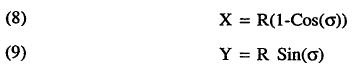where ‘σ’ is equal to one-half of the tool’s total sweep angle. Placing these values into Equation 5 will enable it to be solved for p. After p is known, the values for L, K and J can be found as follows: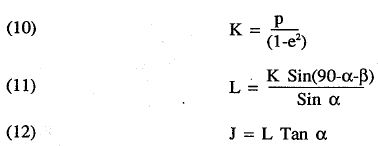If Equation 7 is higher than 1, which would be the case for negative rake tools with a top rake angle less than twice the clearance angle, the ellipse’s minor axis will lie along the tool axis. In this case, the ellipse formula is given as: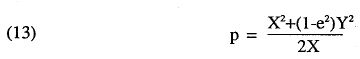In this case, the following equations hold true: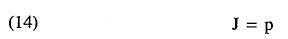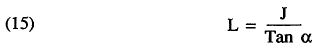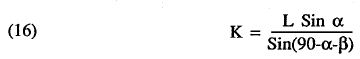If the preferred tool exhibits a cylindrical clearance angle (Figure 3), the major axis of the ellipse will always lie along the tool axis. The calculations are identical to those just discussed, varying only in terms of the calculation of e.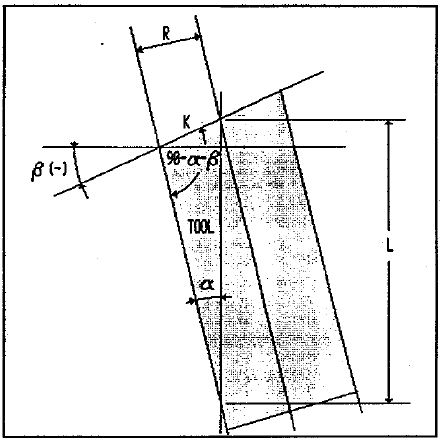Figure 3. A tool with a negative top rake and a cylindrical clearance angle.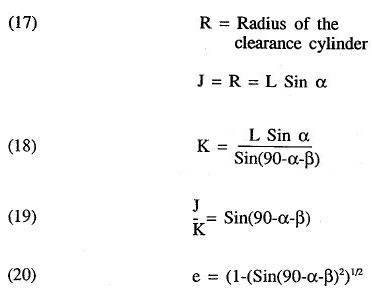Once the value of e has been determined, Equation 5 can be solved for p to find the values for K using Equation 10, and J using Equation 21.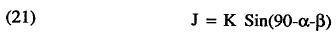Once the ellipse formed by the tool nose has been defined, this ellipse should be projected into the tool’s cutting plane. In order to project the nose of the tool into this plane, The K dimension should be foreshortened by the cosine of the top rake angle, β.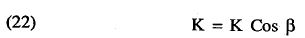Once the projection of the tool shape into the cutting plane is known, an additional point has to be taken into account. For all tools exhibiting a top rake other than zero, the point on the tool which is truly cutting the workpiece lies beyond the cutting plane by an amount which is a function of the instantaneous slope of the work, the tool orientation angle, the slope of the work at its center of rotation, the tool tip dimensions J and K, and the top rake angle. This induces only small errors, but corrections must be built within the tool path to account for the results of this geometry. As the complete treatment of this geometry falls beyond the scope of this discussion, interested users are left to develop a technique that can be applied to resolve these errors.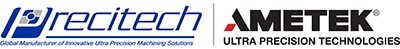This information has been sourced, reviewed and adapted from materials provided by Precitech.

## Citations

• APA

AMETEK Precitech. (2019, December 12). Characterizing the Geometry of Monocrystalline Diamond Contouring Tools. AZoM. Retrieved on September 28, 2020 from https://www.azom.com/article.aspx?ArticleID=12287.

• MLA

AMETEK Precitech. "Characterizing the Geometry of Monocrystalline Diamond Contouring Tools". AZoM. 28 September 2020. <https://www.azom.com/article.aspx?ArticleID=12287>.

• Chicago

AMETEK Precitech. "Characterizing the Geometry of Monocrystalline Diamond Contouring Tools". AZoM. https://www.azom.com/article.aspx?ArticleID=12287. (accessed September 28, 2020).

• Harvard

AMETEK Precitech. 2019. Characterizing the Geometry of Monocrystalline Diamond Contouring Tools. AZoM, viewed 28 September 2020, https://www.azom.com/article.aspx?ArticleID=12287.